# The Real Numbers

29. Oct 2015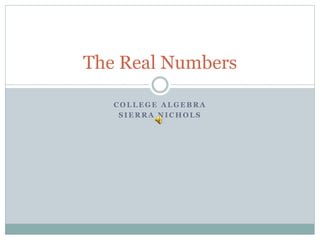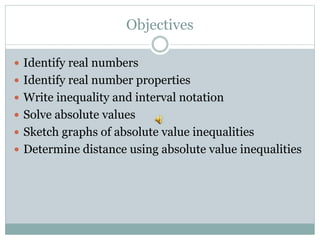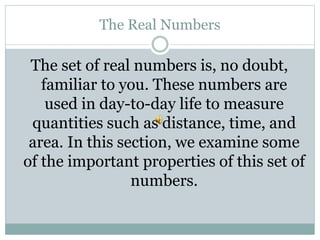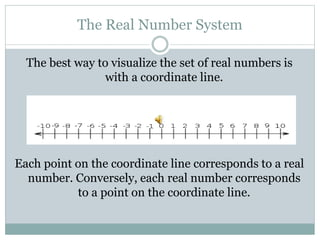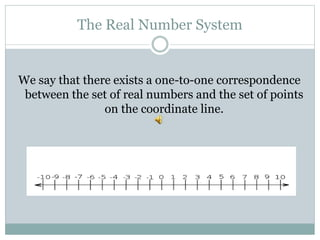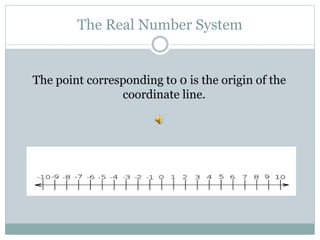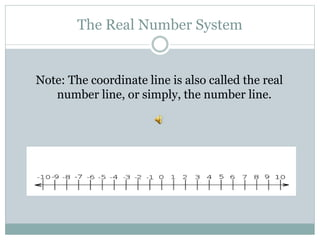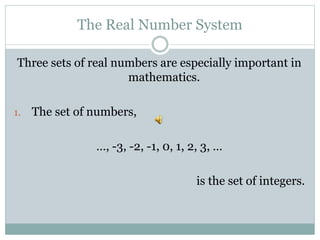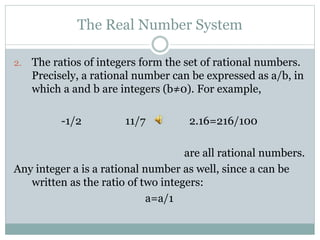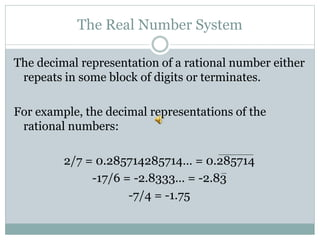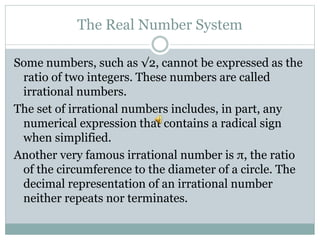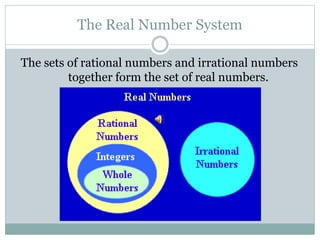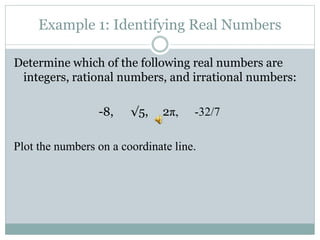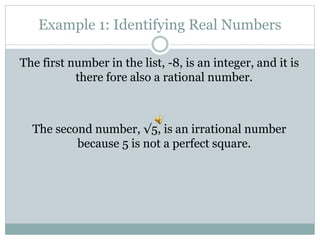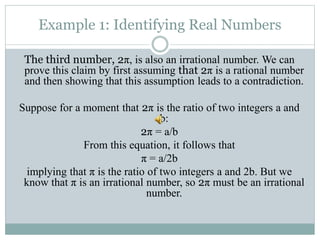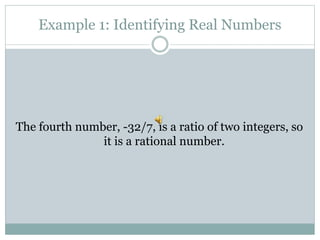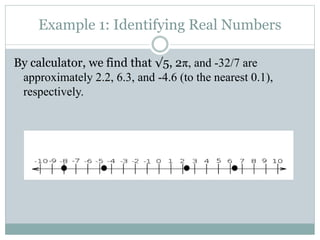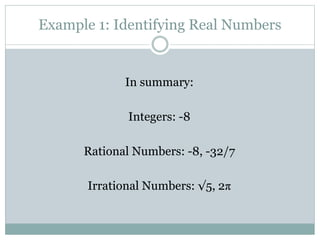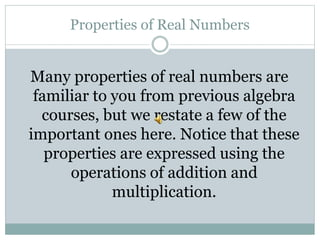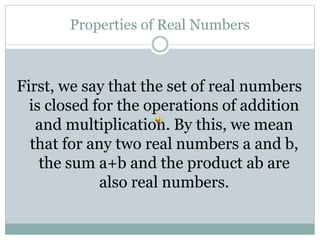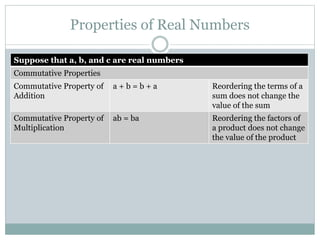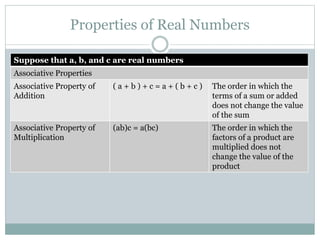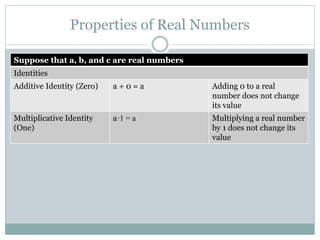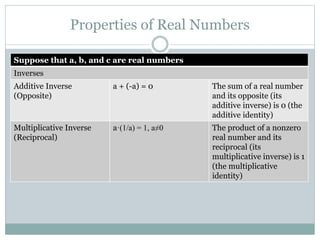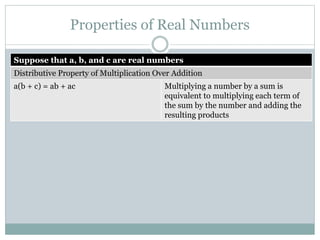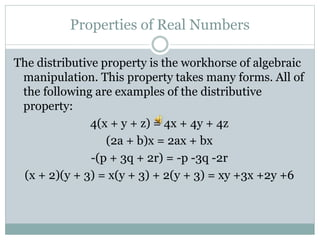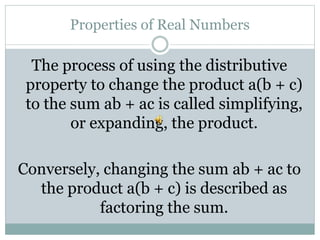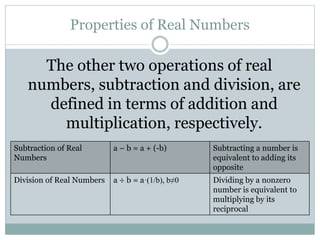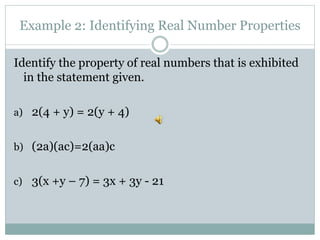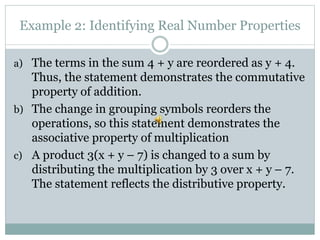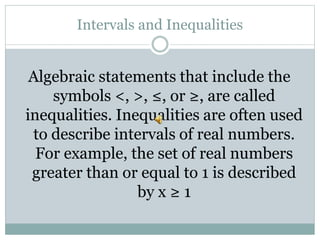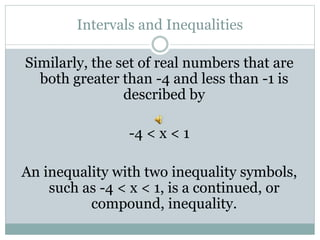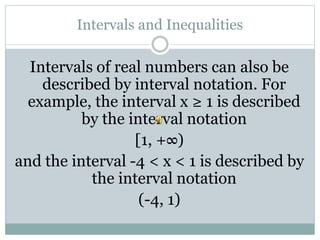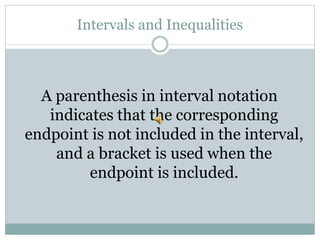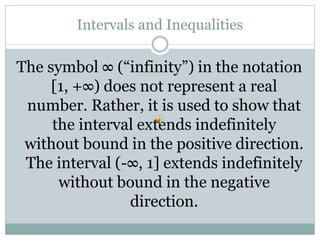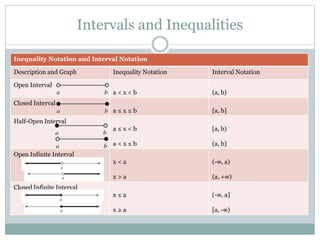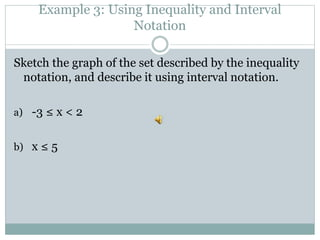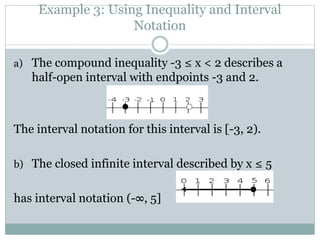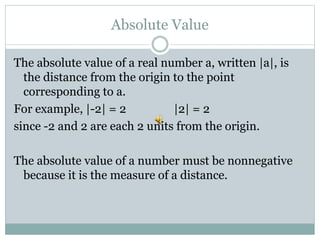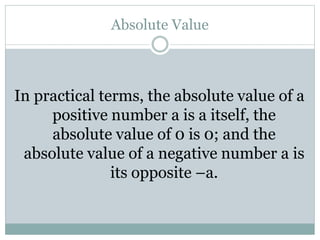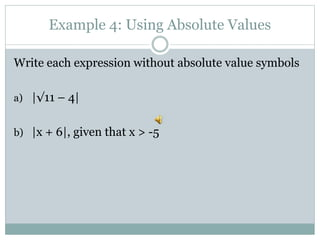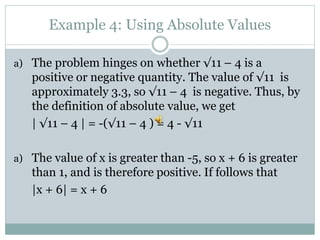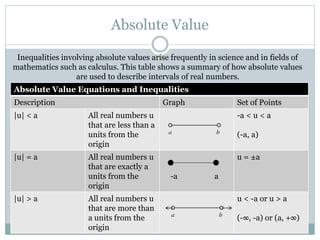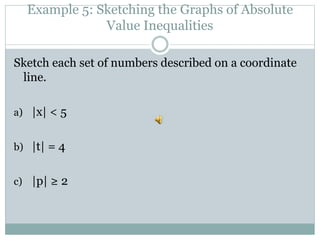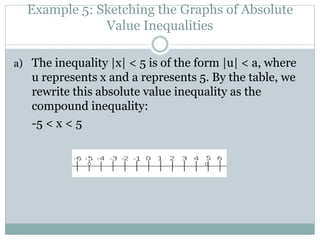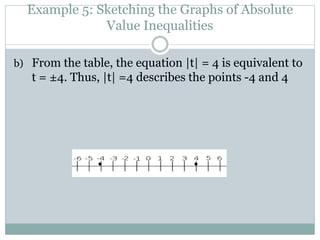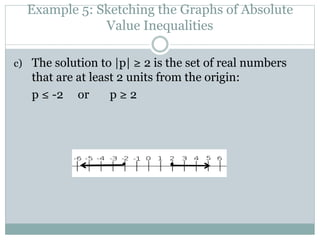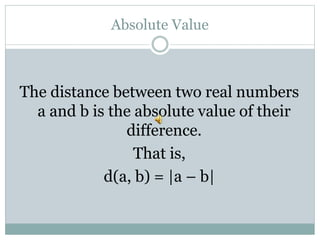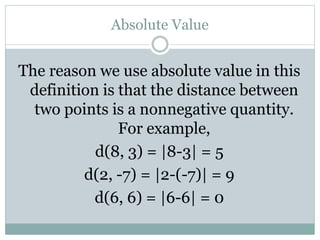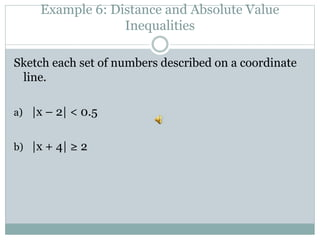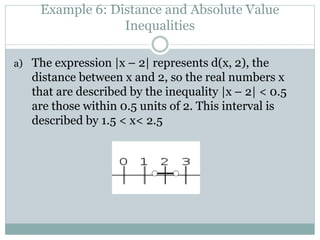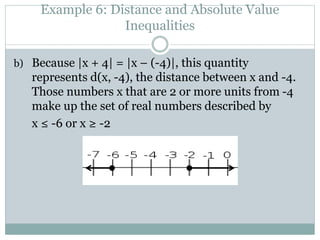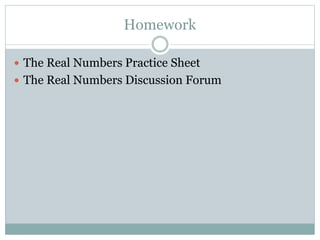1 von 53

### The Real Numbers

• 1. C O L L E G E A L G E B R A S I E R R A N I C H O L S The Real Numbers
• 2. Objectives  Identify real numbers  Identify real number properties  Write inequality and interval notation  Solve absolute values  Sketch graphs of absolute value inequalities  Determine distance using absolute value inequalities
• 3. The Real Numbers The set of real numbers is, no doubt, familiar to you. These numbers are used in day-to-day life to measure quantities such as distance, time, and area. In this section, we examine some of the important properties of this set of numbers.
• 4. The Real Number System The best way to visualize the set of real numbers is with a coordinate line. Each point on the coordinate line corresponds to a real number. Conversely, each real number corresponds to a point on the coordinate line.
• 5. The Real Number System We say that there exists a one-to-one correspondence between the set of real numbers and the set of points on the coordinate line.
• 6. The Real Number System The point corresponding to 0 is the origin of the coordinate line.
• 7. The Real Number System Note: The coordinate line is also called the real number line, or simply, the number line.
• 8. The Real Number System Three sets of real numbers are especially important in mathematics. 1. The set of numbers, …, -3, -2, -1, 0, 1, 2, 3, … is the set of integers.
• 9. The Real Number System 2. The ratios of integers form the set of rational numbers. Precisely, a rational number can be expressed as a/b, in which a and b are integers (b≠0). For example, -1/2 11/7 2.16=216/100 are all rational numbers. Any integer a is a rational number as well, since a can be written as the ratio of two integers: a=a/1
• 10. The Real Number System The decimal representation of a rational number either repeats in some block of digits or terminates. For example, the decimal representations of the rational numbers: 2/7 = 0.285714285714… = 0.285714 -17/6 = -2.8333… = -2.83 -7/4 = -1.75
• 11. The Real Number System Some numbers, such as √2, cannot be expressed as the ratio of two integers. These numbers are called irrational numbers. The set of irrational numbers includes, in part, any numerical expression that contains a radical sign when simplified. Another very famous irrational number is π, the ratio of the circumference to the diameter of a circle. The decimal representation of an irrational number neither repeats nor terminates.
• 12. The Real Number System The sets of rational numbers and irrational numbers together form the set of real numbers.
• 13. Example 1: Identifying Real Numbers Determine which of the following real numbers are integers, rational numbers, and irrational numbers: -8, √5, 2π, -32/7 Plot the numbers on a coordinate line.
• 14. Example 1: Identifying Real Numbers The first number in the list, -8, is an integer, and it is there fore also a rational number. The second number, √5, is an irrational number because 5 is not a perfect square.
• 15. Example 1: Identifying Real Numbers The third number, 2π, is also an irrational number. We can prove this claim by first assuming that 2π is a rational number and then showing that this assumption leads to a contradiction. Suppose for a moment that 2π is the ratio of two integers a and b: 2π = a/b From this equation, it follows that π = a/2b implying that π is the ratio of two integers a and 2b. But we know that π is an irrational number, so 2π must be an irrational number.
• 16. Example 1: Identifying Real Numbers The fourth number, -32/7, is a ratio of two integers, so it is a rational number.
• 17. Example 1: Identifying Real Numbers By calculator, we find that √5, 2π, and -32/7 are approximately 2.2, 6.3, and -4.6 (to the nearest 0.1), respectively.
• 18. Example 1: Identifying Real Numbers In summary: Integers: -8 Rational Numbers: -8, -32/7 Irrational Numbers: √5, 2π
• 19. Properties of Real Numbers Many properties of real numbers are familiar to you from previous algebra courses, but we restate a few of the important ones here. Notice that these properties are expressed using the operations of addition and multiplication.
• 20. Properties of Real Numbers First, we say that the set of real numbers is closed for the operations of addition and multiplication. By this, we mean that for any two real numbers a and b, the sum a+b and the product ab are also real numbers.
• 21. Properties of Real Numbers Suppose that a, b, and c are real numbers Commutative Properties Commutative Property of Addition a + b = b + a Reordering the terms of a sum does not change the value of the sum Commutative Property of Multiplication ab = ba Reordering the factors of a product does not change the value of the product
• 22. Properties of Real Numbers Suppose that a, b, and c are real numbers Associative Properties Associative Property of Addition ( a + b ) + c = a + ( b + c ) The order in which the terms of a sum or added does not change the value of the sum Associative Property of Multiplication (ab)c = a(bc) The order in which the factors of a product are multiplied does not change the value of the product
• 23. Properties of Real Numbers Suppose that a, b, and c are real numbers Identities Additive Identity (Zero) a + 0 = a Adding 0 to a real number does not change its value Multiplicative Identity (One) a·1 = a Multiplying a real number by 1 does not change its value
• 24. Properties of Real Numbers Suppose that a, b, and c are real numbers Inverses Additive Inverse (Opposite) a + (-a) = 0 The sum of a real number and its opposite (its additive inverse) is 0 (the additive identity) Multiplicative Inverse (Reciprocal) a·(1/a) = 1, a≠0 The product of a nonzero real number and its reciprocal (its multiplicative inverse) is 1 (the multiplicative identity)
• 25. Properties of Real Numbers Suppose that a, b, and c are real numbers Distributive Property of Multiplication Over Addition a(b + c) = ab + ac Multiplying a number by a sum is equivalent to multiplying each term of the sum by the number and adding the resulting products
• 26. Properties of Real Numbers The distributive property is the workhorse of algebraic manipulation. This property takes many forms. All of the following are examples of the distributive property: 4(x + y + z) = 4x + 4y + 4z (2a + b)x = 2ax + bx -(p + 3q + 2r) = -p -3q -2r (x + 2)(y + 3) = x(y + 3) + 2(y + 3) = xy +3x +2y +6
• 27. Properties of Real Numbers The process of using the distributive property to change the product a(b + c) to the sum ab + ac is called simplifying, or expanding, the product. Conversely, changing the sum ab + ac to the product a(b + c) is described as factoring the sum.
• 28. Properties of Real Numbers The other two operations of real numbers, subtraction and division, are defined in terms of addition and multiplication, respectively. Subtraction of Real Numbers a – b = a + (-b) Subtracting a number is equivalent to adding its opposite Division of Real Numbers a ÷ b = a·(1/b), b≠0 Dividing by a nonzero number is equivalent to multiplying by its reciprocal
• 29. Example 2: Identifying Real Number Properties Identify the property of real numbers that is exhibited in the statement given. a) 2(4 + y) = 2(y + 4) b) (2a)(ac)=2(aa)c c) 3(x +y – 7) = 3x + 3y - 21
• 30. Example 2: Identifying Real Number Properties a) The terms in the sum 4 + y are reordered as y + 4. Thus, the statement demonstrates the commutative property of addition. b) The change in grouping symbols reorders the operations, so this statement demonstrates the associative property of multiplication c) A product 3(x + y – 7) is changed to a sum by distributing the multiplication by 3 over x + y – 7. The statement reflects the distributive property.
• 31. Intervals and Inequalities Algebraic statements that include the symbols <, >, ≤, or ≥, are called inequalities. Inequalities are often used to describe intervals of real numbers. For example, the set of real numbers greater than or equal to 1 is described by x ≥ 1
• 32. Intervals and Inequalities Similarly, the set of real numbers that are both greater than -4 and less than -1 is described by -4 < x < 1 An inequality with two inequality symbols, such as -4 < x < 1, is a continued, or compound, inequality.
• 33. Intervals and Inequalities Intervals of real numbers can also be described by interval notation. For example, the interval x ≥ 1 is described by the interval notation [1, +∞) and the interval -4 < x < 1 is described by the interval notation (-4, 1)
• 34. Intervals and Inequalities A parenthesis in interval notation indicates that the corresponding endpoint is not included in the interval, and a bracket is used when the endpoint is included.
• 35. Intervals and Inequalities The symbol ∞ (“infinity”) in the notation [1, +∞) does not represent a real number. Rather, it is used to show that the interval extends indefinitely without bound in the positive direction. The interval (-∞, 1] extends indefinitely without bound in the negative direction.
• 36. Intervals and Inequalities Inequality Notation and Interval Notation Description and Graph Inequality Notation Interval Notation Open Interval a < x < b (a, b) Closed Interval a ≤ x ≤ b [a, b] Half-Open Interval a ≤ x < b a < x ≤ b [a, b) (a, b] Open Infinite Interval x < a x > a (-∞, a) (a, +∞) Closed Infinite Interval x ≤ a x ≥ a (-∞, a] [a, -∞)
• 37. Example 3: Using Inequality and Interval Notation Sketch the graph of the set described by the inequality notation, and describe it using interval notation. a) -3 ≤ x < 2 b) x ≤ 5
• 38. Example 3: Using Inequality and Interval Notation a) The compound inequality -3 ≤ x < 2 describes a half-open interval with endpoints -3 and 2. The interval notation for this interval is [-3, 2). b) The closed infinite interval described by x ≤ 5 has interval notation (-∞, 5]
• 39. Absolute Value The absolute value of a real number a, written |a|, is the distance from the origin to the point corresponding to a. For example, |-2| = 2 |2| = 2 since -2 and 2 are each 2 units from the origin. The absolute value of a number must be nonnegative because it is the measure of a distance.
• 40. Absolute Value In practical terms, the absolute value of a positive number a is a itself, the absolute value of 0 is 0; and the absolute value of a negative number a is its opposite –a.
• 41. Example 4: Using Absolute Values Write each expression without absolute value symbols a) |√11 – 4| b) |x + 6|, given that x > -5
• 42. Example 4: Using Absolute Values a) The problem hinges on whether √11 – 4 is a positive or negative quantity. The value of √11 is approximately 3.3, so √11 – 4 is negative. Thus, by the definition of absolute value, we get | √11 – 4 | = -(√11 – 4 ) = 4 - √11 a) The value of x is greater than -5, so x + 6 is greater than 1, and is therefore positive. If follows that |x + 6| = x + 6
• 43. Absolute Value Absolute Value Equations and Inequalities Description Graph Set of Points |u| < a All real numbers u that are less than a units from the origin -a < u < a (-a, a) |u| = a All real numbers u that are exactly a units from the origin -a a u = ±a |u| > a All real numbers u that are more than a units from the origin u < -a or u > a (-∞, -a) or (a, +∞) Inequalities involving absolute values arise frequently in science and in fields of mathematics such as calculus. This table shows a summary of how absolute values are used to describe intervals of real numbers.
• 44. Example 5: Sketching the Graphs of Absolute Value Inequalities Sketch each set of numbers described on a coordinate line. a) |x| < 5 b) |t| = 4 c) |p| ≥ 2
• 45. Example 5: Sketching the Graphs of Absolute Value Inequalities a) The inequality |x| < 5 is of the form |u| < a, where u represents x and a represents 5. By the table, we rewrite this absolute value inequality as the compound inequality: -5 < x < 5
• 46. Example 5: Sketching the Graphs of Absolute Value Inequalities b) From the table, the equation |t| = 4 is equivalent to t = ±4. Thus, |t| =4 describes the points -4 and 4
• 47. Example 5: Sketching the Graphs of Absolute Value Inequalities c) The solution to |p| ≥ 2 is the set of real numbers that are at least 2 units from the origin: p ≤ -2 or p ≥ 2
• 48. Absolute Value The distance between two real numbers a and b is the absolute value of their difference. That is, d(a, b) = |a – b|
• 49. Absolute Value The reason we use absolute value in this definition is that the distance between two points is a nonnegative quantity. For example, d(8, 3) = |8-3| = 5 d(2, -7) = |2-(-7)| = 9 d(6, 6) = |6-6| = 0
• 50. Example 6: Distance and Absolute Value Inequalities Sketch each set of numbers described on a coordinate line. a) |x – 2| < 0.5 b) |x + 4| ≥ 2
• 51. Example 6: Distance and Absolute Value Inequalities a) The expression |x – 2| represents d(x, 2), the distance between x and 2, so the real numbers x that are described by the inequality |x – 2| < 0.5 are those within 0.5 units of 2. This interval is described by 1.5 < x< 2.5
• 52. Example 6: Distance and Absolute Value Inequalities b) Because |x + 4| = |x – (-4)|, this quantity represents d(x, -4), the distance between x and -4. Those numbers x that are 2 or more units from -4 make up the set of real numbers described by x ≤ -6 or x ≥ -2
• 53. Homework  The Real Numbers Practice Sheet  The Real Numbers Discussion Forum